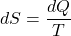# What is the Clausius Inequality?

#### Engineering

To understand what the Clausius Inequality is, one must first look at a thermodynamic system, which exchanges heat with its environment. The Clausius Inequality relates the change in entropy of this thermodynamic system to the heat supplied to the system. Therefore, the Inequality implies a reduction in entropy in the system during the thermodynamic cycle.

In this article, you will learn the Clausius Inequality equation, its derivation, and its applicability to thermodynamic cycles.

## Clausius Inequality Equation

The Clausius Inequality equation highlights the difference between a reversible process and an irreversible process. Therefore, it shows that for an irreversible process, the ratio of the heat entering a thermodynamic system at any point (dQ), to the temperature at the point of heat entry (T) will remain less than or equal to zero for a cycle. Moreover, this ratio represents the change in entropy (dS) of the system.However, for a reversible process (Carnot or ideal cycle), there is no net change in entropy for a complete cycle. Thus, we express the Clausius Inequality equation for this scenario is as follows:## Derivation of Clausius Inequality

The derivation of Clausius Inequality stems from observations of reversible and irreversible processes of a heat engine made by Rudolf Clausius. These processes entail the flow of heat from a high-temperature reservoir into an engine to enable it to work. Then, some of this heat ejects into the environment, which consistutes a low-temperature reservoir. Moreover, he recorded the heat coming into the engine (QH), and the heat leaving the engine into the environment (QL). Also, Clausius recorded the absolute temperature of the high-temperature reservoir (TH), and the absolute temperature of the low temperature reservoir (TL).

### Observation 1

Clausius observed that for a reversible heat engine, the ratio of heat absorbed by the engine to that given off by the engine was consistently equal to the ratio of the high-temperature reservoir and low-temperature reservoir.(1)

### Observation 2

For an irreversible process, Clausius found that the relationship was not the same as in his first observation. Rather, the ratio of heat to absolute temperature increased in the direction of natural heat flow as seen in the second equation.(2)

Practically, because the heat exchange process involves both reversible and irreversible components, both equations combine to become a general one for all cycles.(3)

Finally, integrating the third equation over a cycle gives the Clausius Inequality equation for both reversible and irreversible cycles.## Applicability to Thermodynamic Cycles

The Clausius Inequality gives a mathematical explanation of the second law of thermodynamics. Hence, its application is evident in certain devices that operate in thermodynamic cycles. Also, it is one of the first theorems that clearly addresses the concept of entropy. In addition, it shows the proportional relationship between entropy and energy flow.

As a result, one can confidently state that the transfer of heat will always occur from a hotter body to a cooler body naturally. Therefore, we need to use a device to achieve heat flow from a cooler to a hotter environment.

### Refrigerator

A practical ramification of Clausius’ observation is the use of a compressor in a refrigeration system. The compressor drives the refrigerant to take heat out of the cooler refrigerating space into the hotter environment, through the aid of an evaporator and condenser respectively.